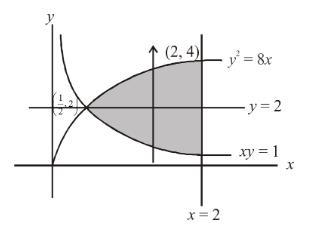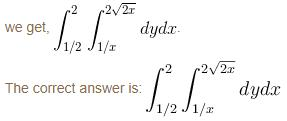Courses

# Integral Calculus MCQ Level - 1

## 10 Questions MCQ Test Topic wise Tests for IIT JAM Physics | Integral Calculus MCQ Level - 1

Description
This mock test of Integral Calculus MCQ Level - 1 for Physics helps you for every Physics entrance exam. This contains 10 Multiple Choice Questions for Physics Integral Calculus MCQ Level - 1 (mcq) to study with solutions a complete question bank. The solved questions answers in this Integral Calculus MCQ Level - 1 quiz give you a good mix of easy questions and tough questions. Physics students definitely take this Integral Calculus MCQ Level - 1 exercise for a better result in the exam. You can find other Integral Calculus MCQ Level - 1 extra questions, long questions & short questions for Physics on EduRev as well by searching above.
QUESTION: 1

### The area of the region bounded by the curves y = |x – 1| and y = 3 – |x| is :

Solution: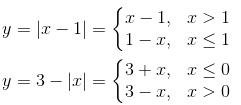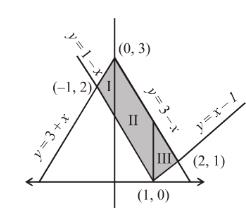The required area can be divided into, three regions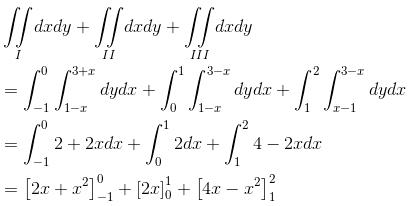= 2 – 1 + 2 + 8 – 4 – 4 +1
= 4 sq. units
The correct answer is: 4 sq. units

QUESTION: 2

### The area enclosed between the curve y = log(x + e) and the coordinate axes is :

Solution:

The area will be given by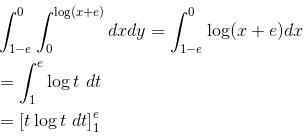e – e + 1
= 1 sq. unit
The correct answer is: 1 sq. unit

QUESTION: 3

### The area of the plane, region bounded by the curves x + 2y2 = 0 and x + 3y2 = 1 is equal to :

Solution:

The required area is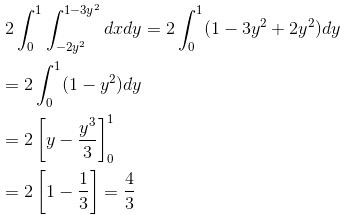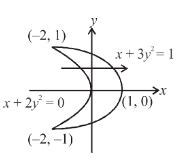The correct answer is 4/3 sq.units

QUESTION: 4

The value of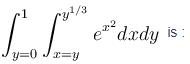Solution:

After changing the order, we get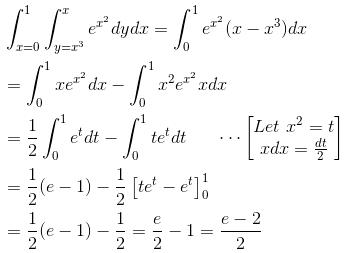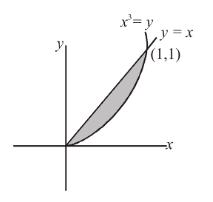The correct answer is: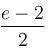QUESTION: 5

The area bounded by the curve y = 2x – x2 and the straight line y = –x is given by :

Solution:

The required area is bounded by the curves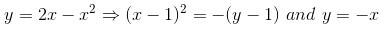∴  The area will be given by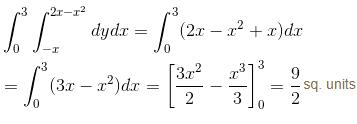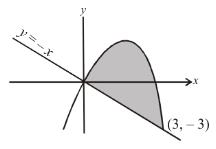The correct answer is:  9/2 sq.units

QUESTION: 6

The change of order of integration of the integral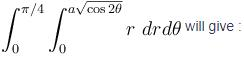Solution:

The region of integration is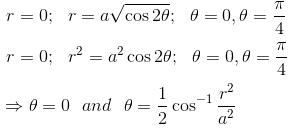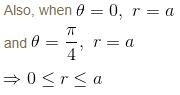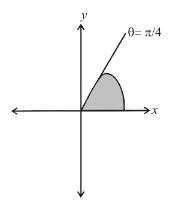Hence, changing the order of integration, we get,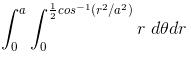The correct answer is: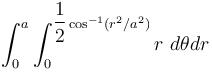QUESTION: 7

The area bounded by the curves |x| + |y| ≥ 1 and x2 + y2 ≤ 1 is :

Solution:

The required area can be evaluated by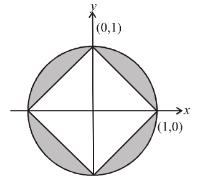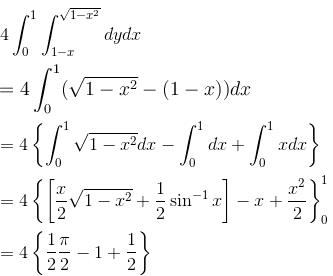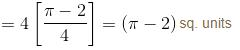Aliter: Required area = Area of circle – Area of square.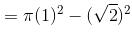= (π - 2)

sq. units

The correct answer is: (π - 2)  sq. units

QUESTION: 8

The area of the region bounded by the curves y = |x – 2|, x = 1, x = 3 and the x–axis is :

Solution:

The required area is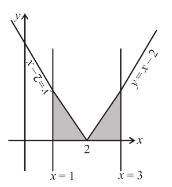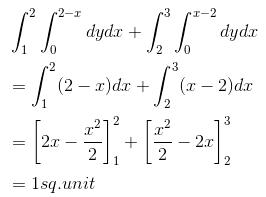The correct answer is: 1 sq. unit

QUESTION: 9

The integral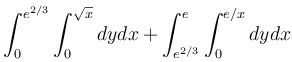becomes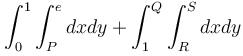after reversing the order of integration.

Then the incorrect option would be :

Solution:

The given region of integration is bounded by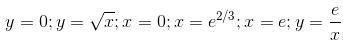plotting all these curves, we have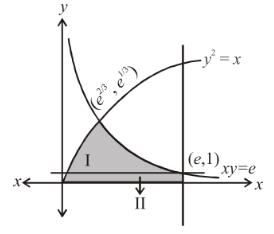Reversing the order of integration, we have the domain of integration divided into 2 regions – I and II.

∴ The integral becomes: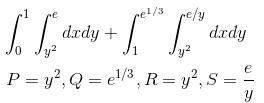The correct answer is:  Q = e

QUESTION: 10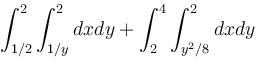is equivalent to :

Solution:

The region of integration is bounded by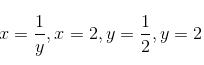and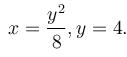Changing the order of integration,